# Progression

-12, 60, -300,1500 need next 2 numbers of pattern

a5 =  -7500
a6 =  37500

### Step-by-step explanation:Did you find an error or inaccuracy? Feel free to write us. Thank you!## Related math problems and questions:

• Probably memberLook at the series 2,6,25,96,285, ? What number should come next?
• NumbersDetermine the number of all positive integers less than 4183444 if each is divisible by 29, 7, 17. What is its sum?
• Gauss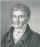Help little C.F. Gauss sum all the integers from 1 to 400.
• SequenceIn the arithmetic sequence is a1=-1, d=4. Which member is equal to the number 203?
• TogetherIf 8 men, 10 women, 16 children collects ₹1024 in 4 days, how many days will be required for 6 men, 5 women and 4 boys to collect ₹768? (₹ is Indian Rupee)
• Three digits numberHow many are three-digit integers such that they no digit repeats?
• Four integersFnd four consecutive integers so that the product of the first two is 70 times smaller than the product of the next two.
• Three ints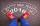The sum of three consecutive integers is 2016. What numbers are they?
• Sum of seriesDetermine the 6-th member and the sum of a geometric series: 5-4/1+16/5-64/25+256/125-1024/625+....
• Geometric sequenceIn the geometric sequence is a4 = 20 a9 = -160. Calculate the first member a1 and quotient q.
• Real estate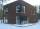The residential house has three entrances numbered by odd numbers arithmetic progression. The sum of the two numbers on the corner entrances is 50. Calculate the highest of these three numbers.
• Geometric progression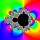Fill 4 numbers between 4 and -12500 to form geometric progression.
• Big numbersHow many natural numbers less than 10 to the sixth can be written in numbers: a) 9.8.7 b) 9.8.0
• Find the sumFind the sum of all natural numbers from 1 and 100, which are divisible by 2 or 5
• Geometric progressionIn geometric progression, a1 = 7, q = 5. Find the condition for n to sum first n members is sn≤217.
• LoanApply for a \$ 59000 loan, the loan repayment period is 8 years, the interest rate 7%. How much should I pay for every month (or every year if paid yearly). Example is for practise geometric progression and/or periodic payment for an annuity.
• Series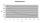Andrew wrote this series of numbers: 1,3,7,15, .... n. Which number in the series does not belong? ?